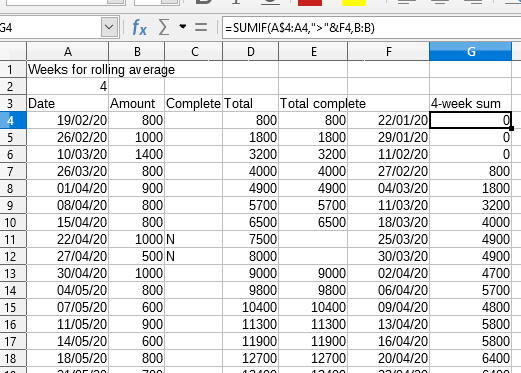# Problem with SUMIF - maybe using dates

I am trying to calculate a rolling average over a date series with irregular dates.

The formula I’m using is `=SUMIF(A\$4:A4,">"&F4,B:B)` where the A column contains the dates in ascending order, the F column contains the date from the A column less the period for the average. But the results aren’t what I’d expect. The first row for column G in the screenshot should say ‘800’ but it doesn’t. It looks as if the column’s shifted down a few cells.Any idea why?

Your `B:B` effectively is `B1:B1048576` so the date criteria matching from A4 on as first row starts to sum at row 1 of column B. You want to start to sum from row 4 as well, so

``````=SUMIF(A\$4:A4;">"&F4;B\$4:B\$9999)
``````

(or whatever max row number instead of 9999).

Thanks @erAck. I thought it would synchronise the two columns.